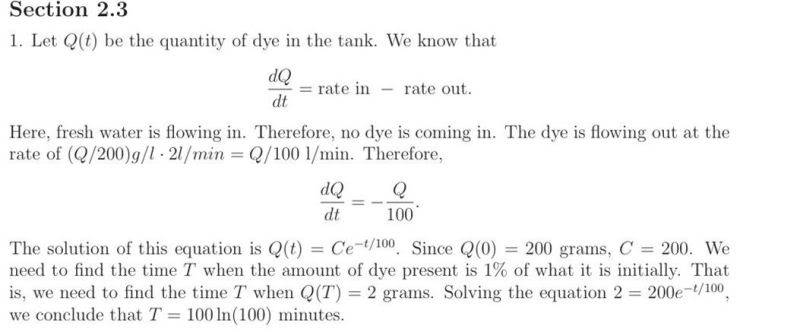# DE: Unit Cancellations Not Making sense

## Homework Statement

Consider a tank used in certain hydrodynamic experiments. After one experiment the tank contains 200 liters of a dye solution with a concentration of 1 g/liter. To prepare for the next experiment, the tank is to be rinsed with fresh water flowing in at a rate of 2 liters/min, the well-stirred solution flowing out at the same rate. Find the time that will elapse before the concentration of dye in the tank reaches 1% of its original value.

## Homework Equations

$$\frac{dQ}{dt}$$=rate in - rate out

## The Attempt at a Solution

Why the heck do they cancel units incorrectly?Dick
Homework Helper
Hard to say until you tell us what the heck Q is.

Q is the amount of dye in the tank.
dQ is the rate of change of the amount of dye in the tank.

Dick